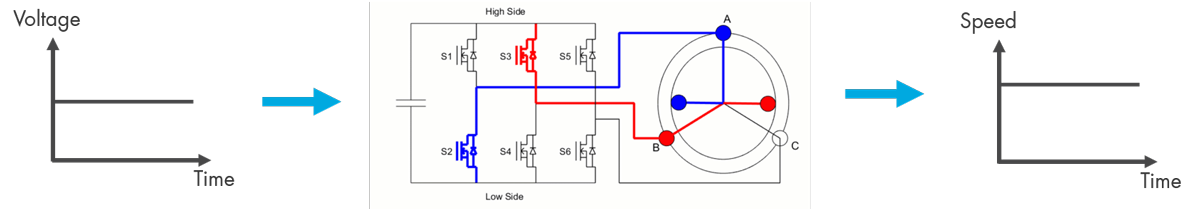# The Mechanics of a BLDC Motor

Consider a motor with three coil windings in the stator and a single pole-pair in the rotor. This type of BLDC motor is driven by six-step commutation or trapezoidal control by a three-phase inverter where the correct phases are commutated every 60 degrees for continuous rotation of the motor. To learn more about six-step commutation and how it works, see the Introduction to Brushless DC Motor Control ebook.

A DC voltage source provides a constant voltage to the three-phase inverter, which converts the DC power to three-phase currents to energize different coil pairs in turn. You can see in the image below that when the applied voltage is constant (plot on the left), the motor turns at a constant speed (plot on the right) due to the proportional relationship between voltage and speed.However, if you want to enable the motor to run at different speeds, you need a controller that will adjust the magnitude of the applied voltage. The following steps outline how to build this algorithm.

## Using Hall Sensors for Sector Detection

First, to control the rotor you have to measure its angular position and speed by using sensors like Hall effect sensors. The Hall sensor doesn’t provide information on precisely where the rotor is within a sector. Instead, it detects when the rotor transitions from one sector to another (see the animation), which is the only input needed to determine when to commutate the motor phases.

What is still missing, however, is the information about which two out of the three phases have to be commutated with each sector crossing. The correct phases are specified by a commutation logic circuit as discussed in the next section.

## Commutating Phases with a Commutation Logic Circuit

The following block diagram shows how different components of the motor control algorithm interact with each other. The commutation logic circuit computes the switching pattern for the three-phase inverter. In the commutation logic table, the letters A, B, and C stand for the three phases of the motor. The high side of the three-phase inverter is labeled with H and the low side with L. If the rotor is within the first sector, the commutation logic selects the top switching pattern, which dictates that the high side switch of phase A and the low side switch of phase C are turned on.

As the rotor transitions to other sectors, the next switching pattern is selected accordingly and sent to the three-phase inverter. In summary, the sensor tells us when to commutate the rotor, and the commutation logic determines the correct phases to energize during each commutation. This way we’re able to spin our motor. The next goal will be to enable the motor to spin at different speeds.

Check out the following videos to learn how to model a three-phase inverter and commutation logic circuit using Simscape Electrical™.